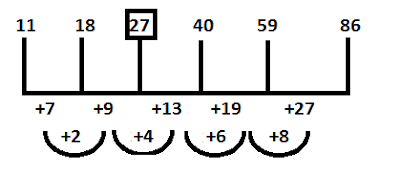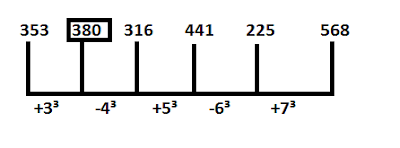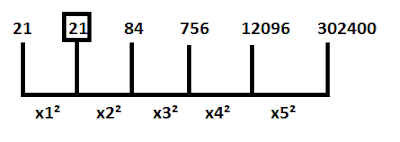# Number Series: Find Wrong Number in the SeriesDirections ( 1-5): In the following number series one number is wrong. Find out the wrong number.

#### Ques 1.

39, 42, 48, 59, 84, 132
1. 132
2. 42
3. 48
4. 59
5. 84

#### Ques 2.

11, 18, 30, 40, 59, 86
1. 30
2. 11
3. 18
4. 59
5. 86

#### Ques 3.

5, 30, 59, 119, 209, 335
1. 59
2. 335
3. 5
4. 119
5. 30

#### Ques 4.

353, 385, 316, 441, 225, 568
1. 568
2. 353
3. 385
4. 225
5. 316

#### Ques 5.

21, 29, 84, 756, 12096, 302400
1. 12096
2. 29
3. 84
4. 756
5. 21

1. 4;
The series isHence, there should be 60 in place of 59.

2. 1;
The series isHence, there should be 27 in place of 30.

3. 5;
The series is
2³ - 3 = 5,      3³ - 4 = 23,     4³-5 = 59
5³-6 = 119,    6³ - 7 = 209,   7³ - 8 = 335
Hence, there should be 23 in place of 30.

4. 3;
The series isHence, there should be 380 in place of 385.

5. 2;
The series isHence, there should be 21 in place of 29.